### Home > CC1 > Chapter 7 > Lesson 7.1.2 > Problem7-23

7-23.
1. Calculate each of the following products. Homework Help ✎

1.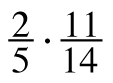2. 12% of 32.8

3. (27.4)(0.02)

4.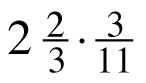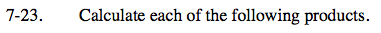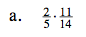$\frac{2}{5}\cdot\frac{11}{14}= \frac{11}{35}$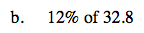0.12(32.8) = ?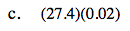Try using a generic rectangle like the ones pictured below.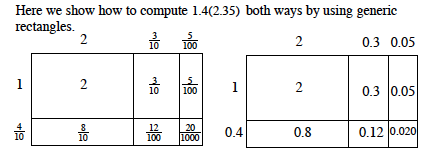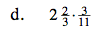This can be written as:

$\left ( \frac{8}{3} \right )\left ( \frac{3}{11} \right )$